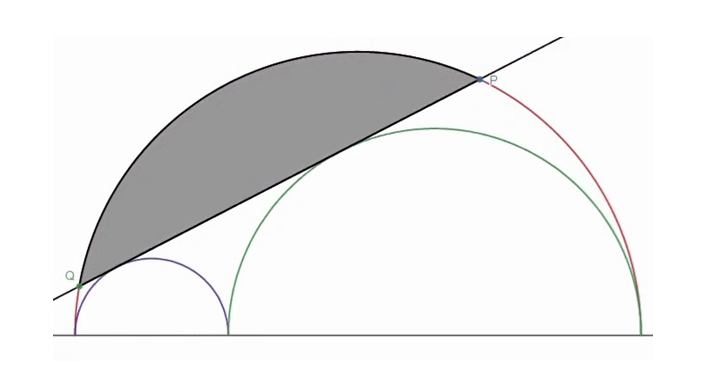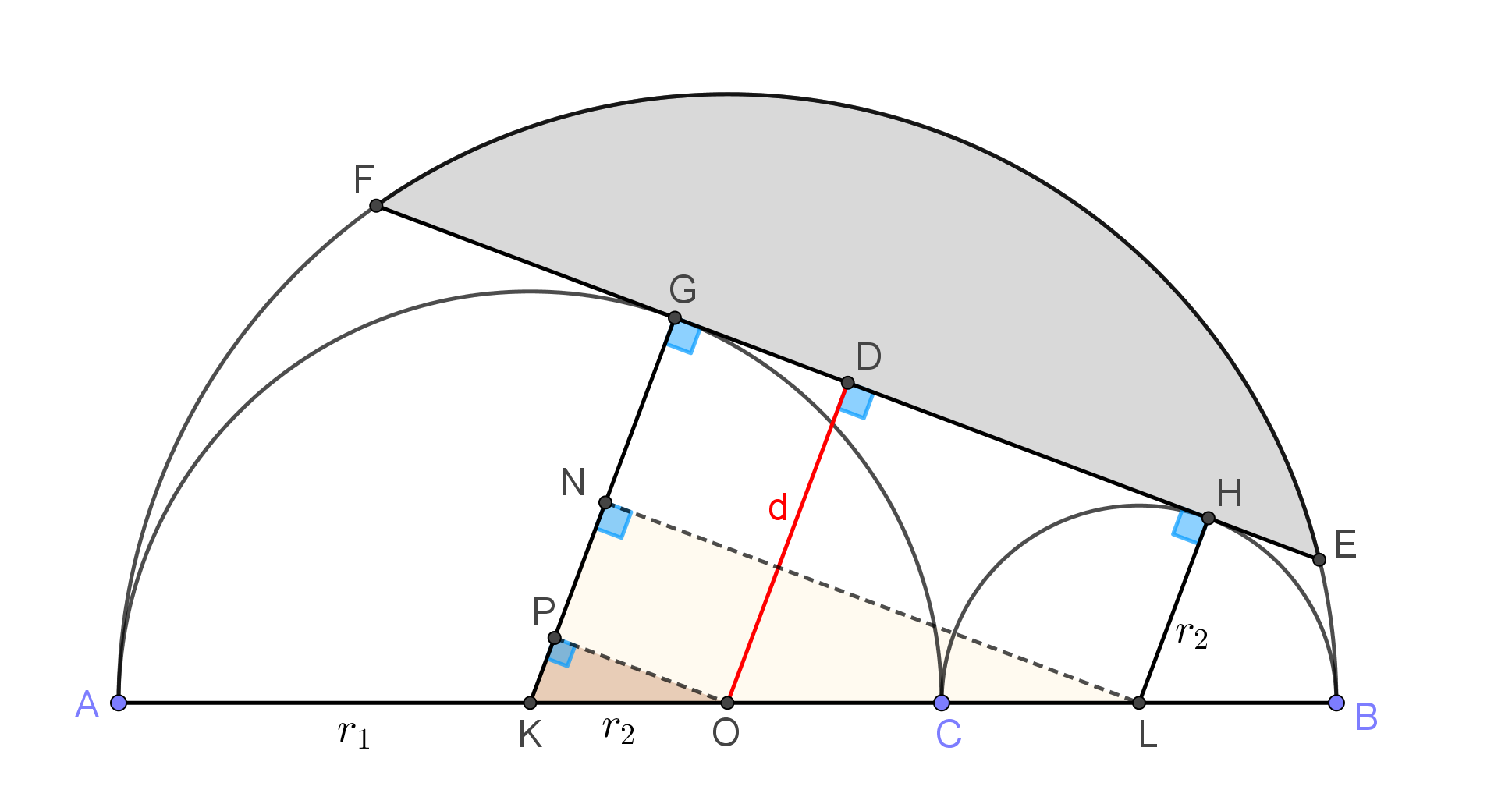# $\Large{\purple{Tangent\space with\space Arbelos}}$

Here is an Arbelos with a tangent drawn to it. I didn't uploaded this because it was larger than $10MB$.$\boxed{\red{Now\space what\space is\space the\space Area\space and\space what\space is\space the\space Maximum\space possible\space Area\space of\space the\space shaded\space region\space if\space the\space Diameter\space of\space the\space bigger\space semicircle\space is\space fixed?}}$

I found the following result :

If $R$ is the radius of the bigger semi-circle and $r_1,r_2$ are the radii of the smaller semi-circles then, $\purple{Area\space of\space the\space shaded\space region}=\dfrac{R^{2}}{2}\cos^{-1}\left(1-\dfrac{8C}{R^{4}}\right)-\dfrac{2\sqrt{C}\sqrt{R^{4}-4C}}{R^{2}}$ $where\space C=\left(R^{2}-r_1r_2\right)r_1r_2$Note by Zakir Husain
3 months, 1 week ago

This discussion board is a place to discuss our Daily Challenges and the math and science related to those challenges. Explanations are more than just a solution — they should explain the steps and thinking strategies that you used to obtain the solution. Comments should further the discussion of math and science.

When posting on Brilliant:

• Use the emojis to react to an explanation, whether you're congratulating a job well done , or just really confused .
• Ask specific questions about the challenge or the steps in somebody's explanation. Well-posed questions can add a lot to the discussion, but posting "I don't understand!" doesn't help anyone.
• Try to contribute something new to the discussion, whether it is an extension, generalization or other idea related to the challenge.

MarkdownAppears as
*italics* or _italics_ italics
**bold** or __bold__ bold
- bulleted- list
• bulleted
• list
1. numbered2. list
1. numbered
2. list
Note: you must add a full line of space before and after lists for them to show up correctly
paragraph 1paragraph 2

paragraph 1

paragraph 2

[example link](https://brilliant.org)example link
> This is a quote
This is a quote
    # I indented these lines
# 4 spaces, and now they show
# up as a code block.

print "hello world"
# I indented these lines
# 4 spaces, and now they show
# up as a code block.

print "hello world"
MathAppears as
Remember to wrap math in $$ ... $$ or $ ... $ to ensure proper formatting.
2 \times 3 $2 \times 3$
2^{34} $2^{34}$
a_{i-1} $a_{i-1}$
\frac{2}{3} $\frac{2}{3}$
\sqrt{2} $\sqrt{2}$
\sum_{i=1}^3 $\sum_{i=1}^3$
\sin \theta $\sin \theta$
\boxed{123} $\boxed{123}$

Sort by:

- 3 months, 1 week ago

Neat problem. How did you arrive at the area of the shaded region? Area of the sector - triangle = Segment?

- 3 months, 1 week ago

The whole Process:

I took the whole case in a coordinate plane with the center of the large semi-circle as the origin and it's diameter as x-axis.

Then the equation of the tangent became : $y=\dfrac{x(r_2-r_1)+r_1^2+t_2^2}{2\sqrt{r_1r_2}}$

Then I found the length of $\overline{PQ}=\dfrac{4\sqrt{R^{2}-r_1r_2}\sqrt{r_1r_2}}{R}$

It is then easier to find the area of the shaded region as you now know the length of the chord...

- 3 months, 1 week ago

- 3 months, 1 week ago

- 3 months, 1 week ago

Using just "elementary" geometry:Let ${{r}_{1}}$ be the radius of the left semicircle and ${{r}_{2}}$ the radius of the right one. WLOG we take ${{r}_{1}}\ge {{r}_{2}}$. Denote by $d=OD$ the apothem of the cord $FE$. Denote by $K$, $O$ and $L$ the centers of the three semicircles as seen in the figure. Let $G$, $H$ be the points of tangency of the cord with the semicircles. Let $LN$ and $OP$ be perpendicular to $KG$. Then we have,

\begin{aligned} & R={{r}_{1}}+{{r}_{2}} \\ & \Rightarrow KO=AO-AK=R-{{r}_{1}}={{r}_{2}} \\ \end{aligned} Since right triangles $PKO$ and $NKL$ are similar, $\frac{PK}{KO}=\frac{NK}{KL}\Rightarrow \frac{{{r}_{1}}-d}{{{r}_{2}}}=\frac{{{r}_{1}}-{{r}_{2}}}{{{r}_{1}}+{{r}_{2}}}\Rightarrow \frac{{{r}_{1}}-d}{{{r}_{2}}}=\frac{{{r}_{1}}-{{r}_{2}}}{R}$ which solves to

$d=\dfrac{1}{R}\left( 2{{r}_{1}}^{2}-2R{{r}_{1}}+{{R}^{2}} \right)$ Now, the area of the circular segment gets maximised when its cord is maximised, which in turn occurs when the apothem $d$ is minimised. The latter happens when ${{r}_{1}}$ equals the $x$-coordinate of the vertex of the parabola $y=\dfrac{1}{R}\left( 2{{x}^{2}}-2Rx+{{R}^{2}} \right)$, i.e. when ${{r}_{1}}=\dfrac{R}{2}$.

In this case, $d=\dfrac{R}{2}$ which is the apothem of the inscribed equilateral triangle, thus $\angle FOE=\dfrac{2\pi }{3}$ and the maximal area of the circular segment is

$A=\dfrac{1}{2}\dfrac{2\pi }{3}{{R}^{2}}-\dfrac{1}{2}{{R}^{2}}\sin \dfrac{2\pi }{3}=\boxed{\left( \dfrac{\pi }{3}-\dfrac{\sqrt{3}}{4} \right){{R}^{2}}}$

$\ \ \$

In general, the area of the circular segment can be expressed as

$A=\dfrac{{{R}^{2}}}{2}{{\cos }^{-1}}\left( 2\dfrac{{{d}^{2}}}{{{R}^{2}}}-1 \right)-d\sqrt{{{R}^{2}}-{{d}^{2}}}$ where $d=\frac{1}{R}\left( 2{{r}_{1}}^{2}-2R{{r}_{1}}+{{R}^{2}} \right)=\frac{1}{R}\left( {{r}_{1}}^{2}+{{r}_{2}}^{2} \right)$

- 3 months, 1 week ago

Very neat solution, I didn't even thought of Apothem!

- 3 months, 1 week ago

I found a mistake in my previous formula so fixed it, now it's absolutely correct

- 3 months, 1 week ago

We can substitute $r_1=x,r_2=R-x$

Then, $Area=\dfrac{R^{2}}{2}\cos^{-1}\left(1-\dfrac{8C}{R^{4}}\right)-\dfrac{2\sqrt{C}\sqrt{R^{4}-4C}}{R^{2}}=\dfrac{R^{2}}{2}\cos^{-1}\left(\dfrac{2d^{2}}{R^{2}}-1\right)-d\sqrt{R^{2}-d^{2}}$ $where\space C=\left(R^{2}-x\left(R-x\right)\right)x\left(R-x\right),d=\dfrac{2x^{2}-2Rx+R^{2}}{R}$ The Graph of the equation looks like this

The maxima of the graph is $x=\dfrac{R}{2}$ (solved by @Thanos Petropoulos ) $\Rightarrow \boxed{A=R^{2}\left(\dfrac{\pi}{3}-\frac{\sqrt{3}}{4}\right)}$

- 3 months, 1 week ago